Journal DetailsFormat
Journal
eISSN
2083-134X
First Published
16 Apr 2011
Publication timeframe
4 times per year
Languages
EnglishOpen Access

# 2D and 3D numerical simulation of fatigue crack growth path and life predictions of a linear elastic

###### Accepted: 07 Oct 2021
Journal DetailsFormat
Journal
eISSN
2083-134X
First Published
16 Apr 2011
Publication timeframe
4 times per year
Languages
English

This paper describes implementation of the finite element method (FEM) to investigate crack growth problems in linear elastic fracture mechanics and the correlation of results with experimental and numerical data. The approach involved using two different software to compute stress intensity factors (SIFs), the crack propagation trajectory, and fatigue life estimation in two and three dimensions. According to the software, crack modeling might be run in various ways. The first is a developed source code program written in the Visual Fortran language, while the second is the widely used ANSYS Mechanical APDL 19.2 software. The fatigue crack propagation trajectory and the corresponding SIFs were predicted using these two software programs. The crack direction was investigated using the maximum circumferential stress theory, and the finite element (FE) analysis for fatigue crack growth was done for both software based on Paris's law. The predicted results in both software demonstrated the influence of holes on the crack growth trajectory and all associated stresses and strains. The study's findings agree with other experimental and numerical crack propagation studies presented in the literature that reveal similar crack propagation trajectory observations.

#### Keywords

Introduction

The prediction of curvilinear fatigue and fracture is a topic for ongoing research. A mathematical study has provided few curved crack path propagation solutions [1, 2], but experimental results can be accurately reproduced in computer ways. The main objective of fracture mechanics is to determine whether or not a structure will fail based on a crack. Crack analysis should start from field stresses in the crack tip, evaluated by the equivalent stress intensity factor (Keq). By comparing the Keq value to a peculiar characteristic of each material called fracture toughness or threshold stress intensity factor, it is possible to determine whether or not a crack member would fail when subjected to static or fatigue loading, respectively. In general, cracks’ initiation and propagation must be associated with stress intensity factors (SIFs) in a complicated state [3,4,5,6,7]. The prediction of curvilinear fatigue and fractures remains a topic of ongoing research. Few curved crack path propagation solutions were obtained by mathematical analysis, but computational approaches can accurately reproduce experimental results [8,9,10]. Numerical simulations typically calculate the energy derivatives in the first order concerning crack length or the equivalent SIFs. Meanwhile, new approaches and methods in several areas of study have been suggested and developed rapidly, including the finite element method (FEM), discrete element method (DEM) [11,12,13], element free Galerkin (EFG) method , extended finite element method (XFEM) [15,16,17,18], cohesive element method [19, 20], and phase-field method . Nevertheless, as a versatile method to simulate crack propagation, the FEM is still prevalent. In general, the propagation of an internal crack in the engineering practice is essential because of its significant effect on the quality and stability of engineering structures. Thus, it is important to predict the crack propagation path for safety and reliability of engineering structures. Therefore, in many industries, the accurate estimation of the crack path and fatigue life estimation is of primary importance in terms of reliability. In various applications, such as aerospace manufacturing and the aviation industry, experimental studies are necessary for fatigue analysis. However, due to high costs, an accurate computational approach is required for crack propagation analysis to prognosticate the direction of crack growth and fatigue life in both static and dynamic loading conditions [22,23,24]. The failure is related to (a) the presence of flaws such as interfaces and cracks and (b) the nature of loads that fluctuate. Cracks tend to initiate and propagate when subjected to fluctuating loads until the structure does not bear the load contributing to complete failure. These cracks are considered to be fatigue cracks, and the expected fatigue life is one of the most significant parameters to determine the structure's safety. It can be computed by adding the number of loading cycles needed to propagate the fatigue crack and lead to failure. The calculation of the growth rate of the crack is generally based on the relationship within the range of SIFs and the crack geometry. One way to predict SIFs is using computational methods such as the XFEM. The XFEM introduced by Belytschko and Black in 1999  was widely used in novel investigations. It is based on the conventional finite element (FE) framework. It uses a novel displacement feature allowing discontinuities to happen, to defeat the need to re-mesh continuously during the crack tip development process . Extended work was offered to promote practical models to estimate the fatigue failure and fatigue growth to subdue fatigue failures. Several experimental tests are proposed [27, 28], but the performance of the experimental procedures is usually time-consuming and costly. An effective way to reduce the laboratory work, time, and costs is to incorporate a simulation methodology to including the numerical analysis. Many fatigue crack problems identified to date by the literature use different computational approaches in simulation for simplistic and complicated geometries in 2D and 3D [10, 22, 29,30,31,32,33,34,35]. In this paper, the ANSYS APDL 19.2 is used to precisely predict the mixed-mode stress intensity and correlated fatigue response for seven specimens of the modified compact tension specimen (MCTS). In particular, three methods were widely used to illustrate the fatigue estimation of different specimens: (a) the fracture mechanics based on Paris and Erdogan ; (b) the method of strain-life independently proposed by Coffin ; and (c) the method of stress-life proposed by Wöhler . The first approach was employed in this study for predicting fatigue life by which the crack tip can be described separately by the SIFs. The primary motivation for this study was to perform a proper comparison of the predicted results for crack propagation parameters using the author's 2D software and the 3D ANSYS software as an alternate tool for simulating fatigue crack growth subjected to mixed-mode loading. The computational time to simulate the crack propagation in the developed 2D program is approximately one-fifth compared with the ANSYS modeling for the same geometry and mesh density.

Mixed mode fatigue life evaluation procedure using ANSYS MECHANICAL APDL 19.2

There are three types of cracks that ANSYS can model: arbitrary, semi-elliptical, and pre-meshed. The Separating Morphing and Adaptive Remeshing Technology (SMART) uses the crack front in the pre-meshed crack approach, and the stress intensity factor is the failure criteria. The rendered node sets will distribute to the front of the crack, as well as to the top and bottom of the crack. By employing SIFs as the failure criteria, the front of the crack is represented in the pre-meshed crack process used by the “Smart Crack Growth” simulation tool. SMART automatically updates the mesh from crack geometry modifications based on crack propagation on each solution level, eliminating the need for long pre-processing sessions. The sphere of influence method around the crack tip that goes through the thickness can be used to improve the mesh around the crack tip. The geometric regions to be described are the crack tip, the crack's top surface, and the crack's bottom surface. Instead, each of these regions is associated with a node-set to be used for analysis. The ANSYS MECHANICAL APDL 19.2 software considers mixed-mode loading where the maximum circumferential stress criterion is implemented. The following is the formula for the direction of crack propagation [32, 33]: $θ=cos−1(3KII2+KIKI2+8KII3KI2+9KII2)$ \theta = {\cos ^{ - 1}}\left( {{{3K_{II}^2 + {K_I}\sqrt {K_I^2 + 8K_{II}^3} } \over {K_I^2 + 9K_{II}^2}}} \right) where KI = Max KI during cyclic loading and KII = Max KII during cyclic loading. The two possibilities for crack growth direction are visualized in Figure 1.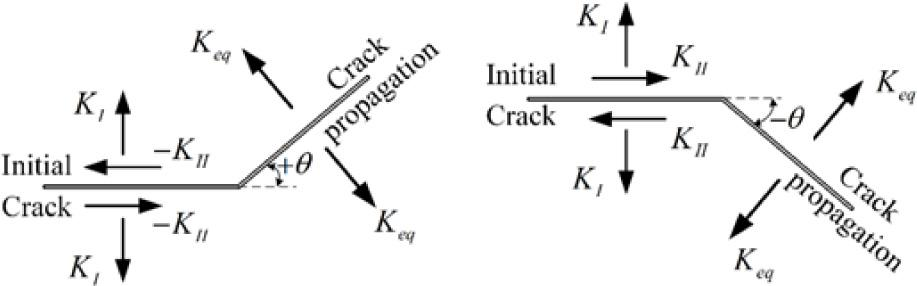Crack propagation angle according to the second mode of SIFs (A) KII > 0 (B) KII < 0.

In ANSYS Mechanical, the simulation of crack propagation is restricted to region II of the typical fatigue crack growth graph based on Paris’ law that can be represented as: $dadN=C(ΔKeq)m$ {{da} \over {dN}} = C{\left( {\Delta {K_{eq}}} \right)^m}

From Eq. (2), for a crack increment, the number of fatigue life cycles may be predicted as: $∫0ΔadaC(ΔKeq)m=∫0ΔNdN=ΔN$ \int\limits_0^{\Delta a} {{{da} \over {C{{\left( {\Delta {K_{eq}}} \right)}^m}}} = } \int\limits_0^{\Delta N} {dN = \Delta N}

The equivalent range for the stress intensity factor formula can be found as follows : $ΔKeq=12cosθ2[(ΔKI(1+cosθ))−3ΔKIIsinθ]$ \Delta {K_{eq}} = {1 \over 2}\cos {\theta \over 2}\left[ {\left( {\Delta {K_I}\left( {1 + \cos \theta } \right)} \right) - 3\Delta {K_{II}}\sin \theta } \right] where ΔKI = the stress intensity factor range in mode I loading and ΔKII = the stress intensity factor range in mode II loading. The ANSYS flow chart is shown in Figure 1.

Developed program procedure

The developed program is a Visual Fortran-based simulation for analyzing two dimensional crack propagation processes under LEFM conditions. This program uses the FE approach to estimate the propagation of quasi-static fatigue cracks in 2D components, taking into account the fracture's mechanical boundary conditions. The adaptive mesh FE method includes four main components for crack trajectory simulation: the mesh optimization technique, the crack criterion, the direction criterion, and the crack propagation strategy. The mesh refinement can be controlled by the characteristic scale of each element, which the error estimator predicts. The step by step analysis is disturbed, and a new FE model is constructed when the error reaches a stated, cumulative error at some point throughout the simulation. Within the initial boundary conditions, the system refines the mesh as needed. After the new mesh is generated, the solution variables (displacement, stresses, strains) are mapped from the previous mesh into the newly created mesh. The analysis is then repeated and continued until the errors exceed the pre-decided number. SIFs are employed in LEFM as fracture criteria. The maximum circumferential stress theory , maximum energy release theory , and minimum strain-energy density theory  are just a few of the methodologies used to predict a crack's propagation direction. The maximum circumferential stress theory has been used in the developed program as a direction criterion.

SIFs method

The most successful engineering implementation of fracture mechanics is crack growth laws based on stress intensity factor ranges . Irwin  defined the stress intensity factor, abbreviated as K, as a mechanical parameter that represents the state of stress at a crack point. For various common crack configurations, many analytical solutions for the SIFs were developed. On the other hand, these analytical solutions are restricted to simple crack geometries and loading conditions . Small-sized yield requirements were implemented , which established a J-integral methodology for investigating the nonlinear behavior of the material . The equivalent domain integral methodology replaces the finite-size domain with a divergence theorem by integration along the contour for FEM. The integral independent contour of the trajectory is as follows : $J=∫C(Wn1−σijnj∂ui∂x)ds$ J = \int\limits_C {\left( {W{n_1} - {\sigma _{ij}}{n_j}{{\partial {u_i}} \over {\partial x}}} \right)ds} where W is the strain-energy density, σij are the stresses, ui is the local i axis displacement, s is the contour arc length, and nj is the outward unit normal to the contour C around the crack tip (Figure 2A).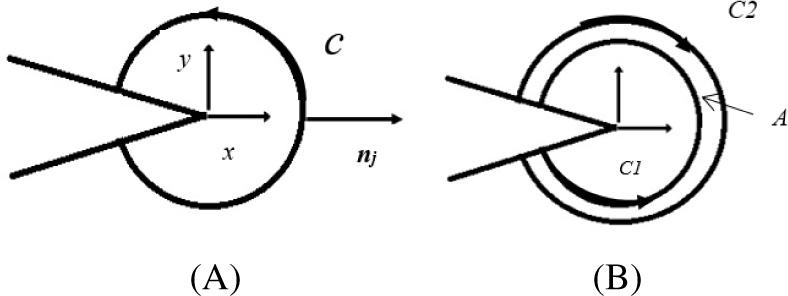(A) Crack tip in an arbitrary contour, (B) J-integral area.

For 2D structures, the contour integral i is replaced by area integral (Figure 2B). Then, Eq. (5) is modified as: $Jk=−∫A[W∂q∂x−σij∂ui∂x∂q∂x]dA−∫A[∂W∂x−∂∂x(σij∂ui∂x)]qdA−∫sti∂ui∂xqds$ \matrix{ {{J_k}} \hfill & = \hfill & { - \int\limits_A {\left[ {W{{\partial q} \over {\partial x}} - {\sigma _{ij}}{{\partial {u_i}} \over {\partial x}}{{\partial q} \over {\partial x}}} \right]dA} } \hfill \cr {} \hfill & {} \hfill & { - \int\limits_A {\left[ {{{\partial W} \over {\partial x}} - {\partial \over {\partial x}}\left( {{\sigma _{ij}}{{\partial {u_i}} \over {\partial x}}} \right)} \right]qdA} - \int\limits_s {{t_i}{{\partial {u_i}} \over {\partial x}}qds} } \hfill \cr }

The step by step procedure of the developed program software is illustrated in Figure 3. The details of these steps are explained in the literature [29, 45, 47,48,49,50]. The adaptive mesh refinement was explained in detail by Bashiri and Alshoaibi .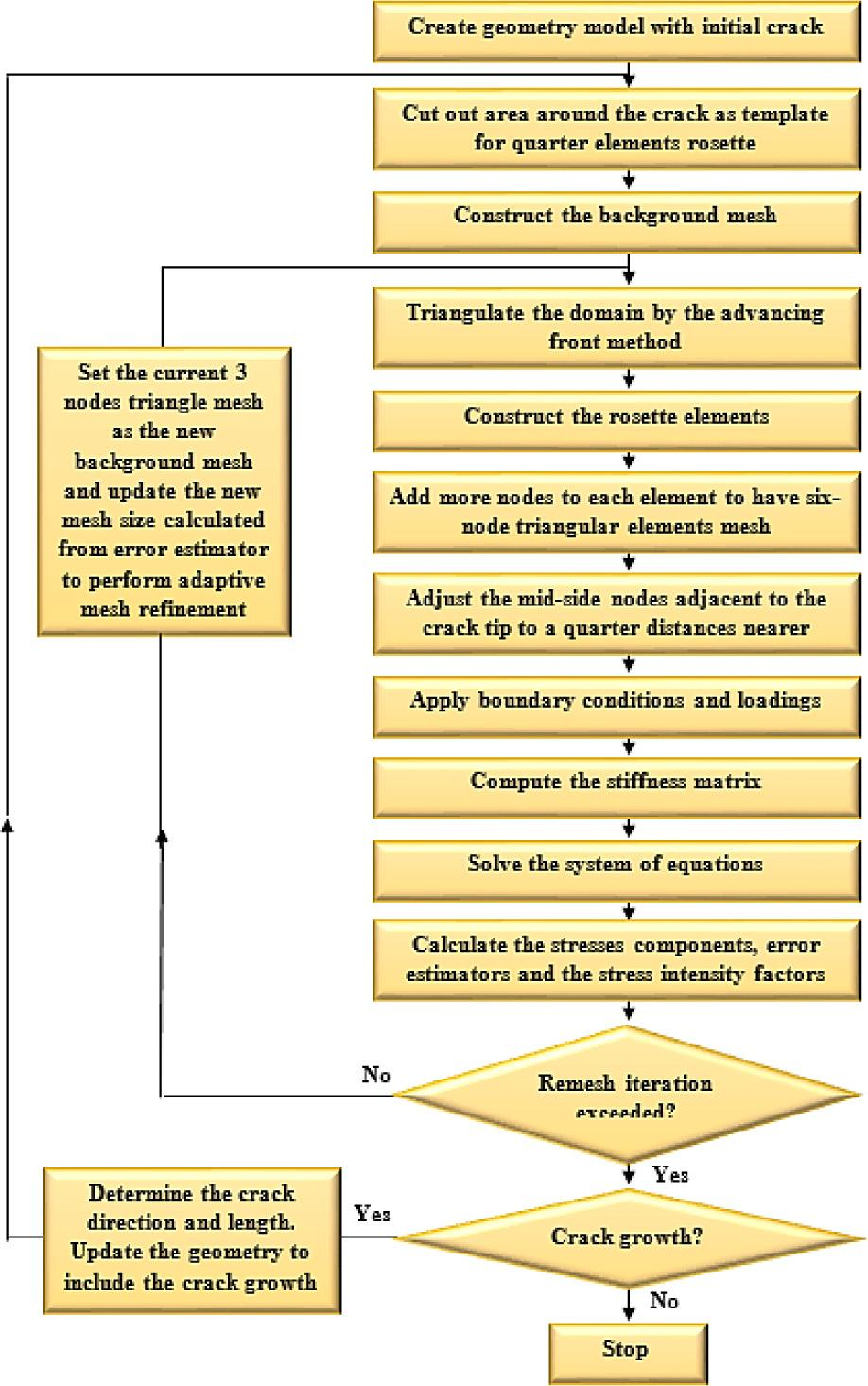Computational procedure of the fatigue crack growth developed program.

In LEFM simulation, the J-integral by definition takes into account translational mechanical energy balance in the tip region of the crack along x axis. Eq. (6) simplifies the computation of mode I and II of SIFs but they do not compute KI and KII separately for multiple load conditions. In these cases, the following integral should be considered : $Jk=−∫A[W∂q∂xk−σij∂ui∂xk∂q∂xj]dA−∫A[W∂q∂xk−σij∂∂xj(∂ui∂xk)]qdA−∫sti∂ui∂xkqds$ \matrix{ {{J_k}} \hfill & = \hfill & { - \int\limits_A {\left[ {W{{\partial q} \over {\partial {x_k}}} - {\sigma _{ij}}{{\partial {u_i}} \over {\partial {x_k}}}{{\partial q} \over {\partial {x_j}}}} \right]dA} } \hfill \cr {} \hfill & {} \hfill & { - \int\limits_A {\left[ {W{{\partial q} \over {\partial {x_k}}} - {\sigma _{ij}}{\partial \over {\partial {x_j}}}\left( {{{\partial {u_i}} \over {\partial {x_k}}}} \right)} \right]} qdA} \hfill \cr {} \hfill & {} \hfill & { - \int\limits_s {{t_i}{{\partial {u_i}} \over {\partial {x_k}}}qds} } \hfill \cr } where k represents the crack tip's index. The SIFs can be calculated using one of the following methods. The first method uses the J-integral and SIFs as: $J1=κ+18μ(KI2+KII2)J2=−κ+14μKIKII$ \matrix{ {{J_1}} \hfill & = \hfill & {{{\kappa + 1} \over {8\mu }}\left( {K_I^2 + K_{II}^2} \right)} \hfill \cr {{J_2}} \hfill & = \hfill & { - {{\kappa + 1} \over {4\mu }}{K_I}{K_{II}}} \hfill \cr }

The second method uses the relation between SIFs and J1 and J2 as: $KI=0.58μκ+1+(J1−J2+J1+J2)KII=0.58μκ+1+(J1−J2−J1+J2)$ \matrix{ {{K_I}} \hfill & = \hfill & {0.5\sqrt {{{8\mu } \over {\kappa + 1}}} + \left( {\sqrt {{J_1} - {J_2}} + \sqrt {{J_1} + {J_2}} } \right)} \hfill \cr {{K_{II}}} \hfill & = \hfill & {0.5\sqrt {{{8\mu } \over {\kappa + 1}}} + \left( {\sqrt {{J_1} - {J_2}} - \sqrt {{J_1} + {J_2}} } \right)} \hfill \cr }

Where κ is the elastic parameter defined as: $κ=|(3−4ν)(3−ν)/(1+ν)plane stressplane strain$ \kappa = \left| {\matrix{ {\left( {3 - 4\nu } \right)} \cr {\left( {3 - \nu } \right)/\left( {1 + \nu } \right)} \cr } \matrix{ {{\rm{plane}}\,{\rm{stress}}} \hfill \cr {{\rm{plane}}\,{\rm{strain}}} \hfill \cr } } \right.

Adaptive mesh refinement is an optimization method used in the field of FE mesh. The a posteriori error estimation used in this scheme is derived from the previous mesh generation. The stress error norm, also known as relative stress norm error, is used to estimate the mesh refinement error. The ratio of element norm stress error to the average standard stress error of the entire region was calculated using h-type adaptive mesh optimization. The mesh size for each element in this scheme is provided as: $he=2Ae,$ {h_e} = \sqrt {2{A_e}}, where Ae is the triangle element area. For each element, the norm stress error is represented by: $‖e‖e2=∫Ωe(σ−σ*)T(σ−σ*)2dΩ=∫Ωe({σxσyτxyσz}−{σx*σy*τxy*σz*})T({σxσyτxyσz}−{σx*σy*τxy*σz*})2dΩ$ \matrix{ {\left\| e \right\|_e^2} \hfill & = \hfill & {\int_{{\Omega ^e}} {{{\left( {\sigma - {\sigma ^*}} \right)}^T}\left( {\sigma - {\sigma ^*}} \right)2{\rm{d}}\Omega } } \hfill \cr {} \hfill & = \hfill & {\int_{{\Omega ^e}} {{{\left( {\left\{ {\matrix{ {{\sigma _x}} \cr {{\sigma _y}} \cr {{\tau _{xy}}} \cr {{\sigma _z}} \cr } } \right\} - \left\{ {\matrix{ {\sigma _x^*} \cr {\sigma _y^*} \cr {\tau _{xy}^*} \cr {\sigma _z^*} \cr } } \right\}} \right)}^T}\left( {\left\{ {\matrix{ {{\sigma _x}} \cr {{\sigma _y}} \cr {{\tau _{xy}}} \cr {{\sigma _z}} \cr } } \right\} - \left\{ {\matrix{ {\sigma _x^*} \cr {\sigma _y^*} \cr {\tau _{xy}^*} \cr {\sigma _z^*} \cr } } \right\}} \right)2{\rm{d}}\Omega } } \hfill \cr }

However, the average norm stress error over the whole domain is represented as: $‖e^‖2=1m∑e=1m∫ΩeσTσ 2dΩ=1m∑e=1m∫Ωe{σxσyτxyσz}T{σxσyτxyσz}2dΩ$ \matrix{ {{{\left\| {\hat {\rm e}} \right\|}^2}} \hfill & = \hfill & {{1 \over m}\sum\limits_{e = 1}^m {\int_{{\Omega ^e}} {{\sigma ^T}\sigma \,2{\rm{d}}\Omega } } } \hfill \cr {} \hfill & = \hfill & {{1 \over m}\sum\limits_{e = 1}^m {\int_{{\Omega ^e}} {{{\left\{ {\matrix{ {{\sigma _x}} \cr {{\sigma _y}} \cr {{\tau _{xy}}} \cr {{\sigma _z}} \cr } } \right\}}^{\rm{T}}}\left\{ {\matrix{ {{\sigma _x}} \cr {{\sigma _y}} \cr {{\tau _{xy}}} \cr {{\sigma _z}} \cr } } \right\}2{\rm{d}}\Omega } } } \hfill \cr } where m is the overall number of elements in the entire domain and σ* is the smoothed stress vector. The integration with the triangular isoparametric dimension will be converted in the FEM by the summation of quadratics according to the Radau rules as follows: $‖e‖e2=∫−11∫−11({σ(ξ, η)xσ(ξ, η)yτ(ξ, η)xyσ(ξ, η)z}−{σ(ξ, η)x*σ(ξ, η)y*τ(ξ, η)xy*σ(ξ, η)z*})T({σ(ξ, η)xσ(ξ, η)yτ(ξ, η)xyσ(ξ, η)z}−{σ(ξ, η)x*σ(ξ, η)y*τ(ξ, η)xy*σ(ξ, η)z*})te det Jedξdη=∑p=13({σ(ξp, ηp)xσ(ξp, ηp)yτ(ξp, ηp)xyσ(ξp, ηp)z}−{σ(ξp, ηp)x*σ(ξp, ηp)y*τ(ξp, ηp)xy*σ(ξp, ηp)z*})T({σ(ξp, ηp)xσ(ξp, ηp)yτ(ξp, ηp)xyσ(ξp, ηp)z}−{σ(ξp, ηp)x*σ(ξp, ηp)y*τ(ξp, ηp)xy*σ(ξp, ηp)z*})te det JeWp$ \matrix{ {\left\| {\rm{e}} \right\|_e^2} \hfill & = \hfill & {\int\limits_{ - 1}^1 {\int\limits_{ - 1}^1 {{{\left( {\left\{ {\matrix{ {\sigma {{\left( {\xi ,\,\eta } \right)}_x}} \cr {\sigma {{\left( {\xi ,\,\eta } \right)}_y}} \cr {\tau {{\left( {\xi ,\,\eta } \right)}_{xy}}} \cr {\sigma {{\left( {\xi ,\,\eta } \right)}_z}} \cr } } \right\} - \left\{ {\matrix{ {\sigma \left( {\xi ,\,\eta } \right)_x^*} \cr {\sigma \left( {\xi ,\,\eta } \right)_y^*} \cr {\tau \left( {\xi ,\,\eta } \right)_{xy}^*} \cr {\sigma \left( {\xi ,\,\eta } \right)_z^*} \cr } } \right\}} \right)}^{\rm{T}}}} } } \hfill \cr {} \hfill & {} \hfill & {\left( {\left\{ {\matrix{ {\sigma {{\left( {\xi ,\,\eta } \right)}_x}} \cr {\sigma {{\left( {\xi ,\,\eta } \right)}_y}} \cr {\tau {{\left( {\xi ,\,\eta } \right)}_{xy}}} \cr {\sigma {{\left( {\xi ,\,\eta } \right)}_z}} \cr } } \right\} - \left\{ {\matrix{ {\sigma \left( {\xi ,\,\eta } \right)_x^*} \cr {\sigma \left( {\xi ,\,\eta } \right)_y^*} \cr {\tau \left( {\xi ,\,\eta } \right)_{xy}^*} \cr {\sigma \left( {\xi ,\,\eta } \right)_z^*} \cr } } \right\}} \right){t^e}\,\det \,{J^e}d\xi d\eta } \hfill \cr {} \hfill & = \hfill & {{{\sum\limits_{p = 1}^3 {\left( {\left\{ {\matrix{ {\sigma {{\left( {{\xi _p},\,{\eta _p}} \right)}_x}} \cr {\sigma {{\left( {{\xi _p},\,{\eta _p}} \right)}_y}} \cr {\tau {{\left( {{\xi _p},\,{\eta _p}} \right)}_{xy}}} \cr {\sigma {{\left( {{\xi _p},\,{\eta _p}} \right)}_z}} \cr } } \right\} - \left\{ {\matrix{ {\sigma \left( {{\xi _p},\,{\eta _p}} \right)_x^*} \cr {\sigma \left( {{\xi _p},\,{\eta _p}} \right)_y^*} \cr {\tau \left( {{\xi _p},\,{\eta _p}} \right)_{xy}^*} \cr {\sigma \left( {{\xi _p},\,{\eta _p}} \right)_z^*} \cr } } \right\}} \right)} }^{\rm{T}}}} \hfill \cr {} \hfill & {} \hfill & {\left( {\left\{ {\matrix{ {\sigma {{\left( {{\xi _p},\,{\eta _p}} \right)}_x}} \cr {\sigma {{\left( {{\xi _p},\,{\eta _p}} \right)}_y}} \cr {\tau {{\left( {{\xi _p},\,{\eta _p}} \right)}_{xy}}} \cr {\sigma {{\left( {{\xi _p},\,{\eta _p}} \right)}_z}} \cr } } \right\} - \left\{ {\matrix{ {\sigma \left( {{\xi _p},\,{\eta _p}} \right)_x^*} \cr {\sigma \left( {{\xi _p},\,{\eta _p}} \right)_y^*} \cr {\tau \left( {{\xi _p},\,{\eta _p}} \right)_{xy}^*} \cr {\sigma \left( {{\xi _p},\,{\eta _p}} \right)_z^*} \cr } } \right\}} \right){t^e}\,\det \,{J^e}{W_p}} \hfill \cr } and similarly $‖e^‖2=1m∑e=1m∑p=13({σ(ξp, ηp)xσ(ξp, ηp)yτ(ξp, ηp)xyσ(ξp, ηp)z})T({σ(ξp, ηp)xσ(ξp, ηp)yτ(ξp, ηp)xyσ(ξp, ηp)z})tedetJeWp$ \matrix{ {{{\left\| {{\rm{\hat e}}} \right\|}^2}} \hfill & = \hfill & {{1 \over m}\sum\limits_{e = 1}^m {\sum\limits_{p = 1}^3 {{{\left( {\left\{ {\matrix{ {\sigma {{\left( {{\xi _p},\,{\eta _p}} \right)}_x}} \cr {\sigma {{\left( {{\xi _p},\,{\eta _p}} \right)}_y}} \cr {\tau {{\left( {{\xi _p},\,{\eta _p}} \right)}_{xy}}} \cr {\sigma {{\left( {{\xi _p},\,{\eta _p}} \right)}_z}} \cr } } \right\}} \right)}^{\rm{T}}}} } } \hfill \cr {} \hfill & {} \hfill & {\left( {\left\{ {\matrix{ {\sigma {{\left( {{\xi _p},\,{\eta _p}} \right)}_x}} \cr {\sigma {{\left( {{\xi _p},\,{\eta _p}} \right)}_y}} \cr {\tau {{\left( {{\xi _p},\,{\eta _p}} \right)}_{xy}}} \cr {\sigma {{\left( {{\xi _p},\,{\eta _p}} \right)}_z}} \cr } } \right\}} \right){t^e}\det {J^e}{W_p}} \hfill \cr } where te is the thickness of the element for a plane stress case and te = 1 is the plane strain case. Consequently, the relative stress norm error ζe for each item is considerably <5%, which is acceptable for many applications in engineering. Thus, $ζe=‖e‖e‖e^‖≤ζ$ {\zeta _e} = {{{{\left\| e \right\|}_e}} \over {\left\| {\hat e} \right\|}} \le \zeta and the new element relative stress error level is identified as a permissible error by: {\varepsilon _e} = {{{{\left\| e \right\|}_e}} \over {\zeta \left\| {\hat e} \right\|}} \le 1

This means each element with ɛe is required to be refined and the new size of the mesh needs to be expected. Here the asymptotic convergence rate criteria are implemented, which presume: $‖e‖e∝hep$ {\left\| e \right\|_e} \propto h_e^p where p is the approximation of polynomial order. In this study, p = 2 since the quadratic polynomial is used for the calculation of FEs. Thus, the new element estimated size is: $hN=1εehe$ {h_N} = {1 \over {\sqrt {{\varepsilon _e}} }}{h_e}

Depending on the user numbers of refinement, the existing mesh will be considered as the new background mesh and the advancing front method will be replicated. The element type was triangular with six nodes.

Results and discussion
Cracked plate with four holes

As illustrated in Figure 4, a four holes’ rectangular plate of 10 mm diameter and a 6 mm long edge crack is analyzed. The dimensions of the plate are 100 mm × 100 mm × 10 mm. The plate is supported on the bottom and is subjected to a tension force of 10 MPa. The plate is made of aluminium 7075-T6, whose properties are shown in Table 1.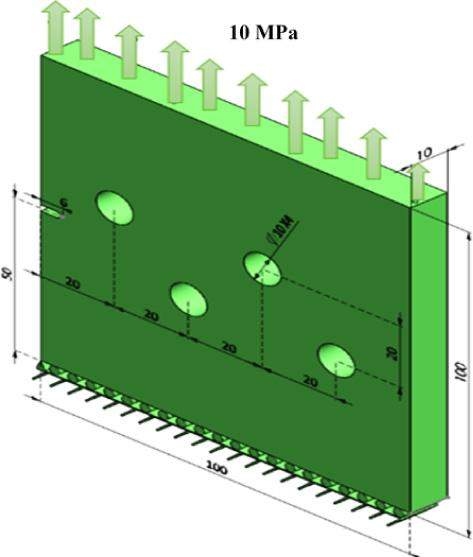Cracked plate with four holes and one edge crack.

Mechanical properties of aluminium 7075-T6 

Property Value in metric unit
Modulus of elasticity, E 72 GPa
Poisson's ratio, υ 0.33
Yield strength, σy 469 MPa
Ultimate strength, σu 538 MPa
Fracture toughness, KIC 3,288.76 MPa $mm$ \sqrt {mm}

The geometry is fixed in the x and y directions at the bottom face in the developed program, while in Ansys the geometry is fixed in the x, y, and z directions.

The meshed geometry created in Ansys workbench has 14,737 nodes and 7,177 elements, while the meshed geometry generated in the developed program has 3,933 nodes and 1,911 elements, as represented in Figure 5.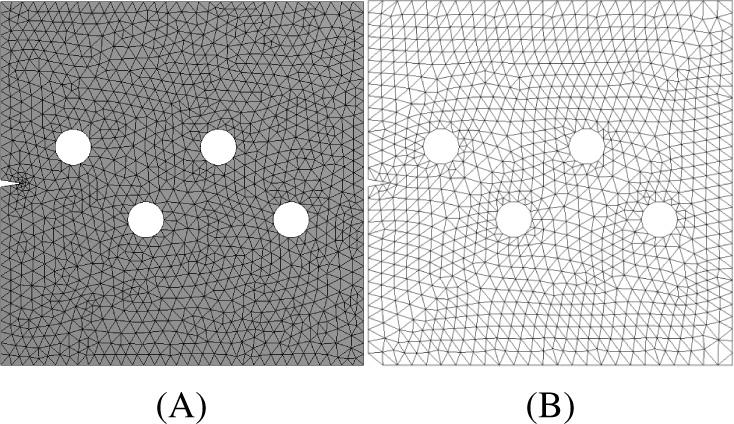Initial generated mesh (A) Ansys Workbench, (B) developed program.

The predicted crack growth paths in both software is shown in Figure 6, and these were also in good agreement with that path predicted by Knowles and Sternberg  using the fast multipole method (FMM) boundary element method.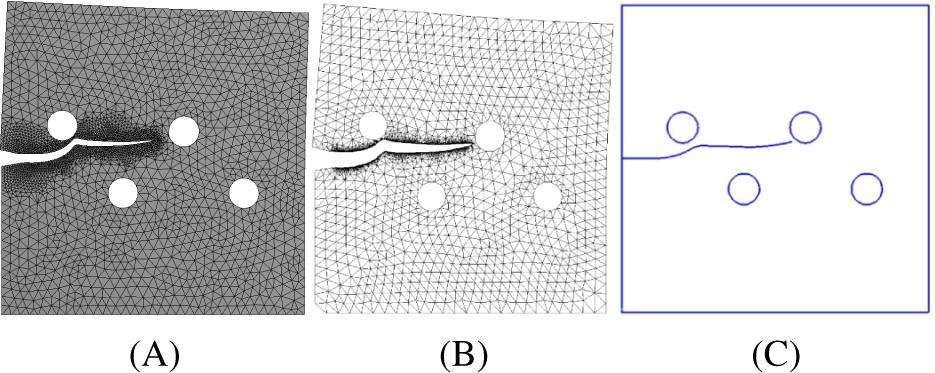Crack growth path: (A) Ansys Workbench, (B) developed program, (C) FMM backscattered electron macroscope (BEM) . FMM, fast multipole method.

The maximum and minimum principal stresses were compared side by side in both programs, as represented in Figure 7. In Figure 7, the crack starts to grow in a straight line as there is no influence of any holes. As it approaches the hole, the path propagates towards the hole. The crack deviated its direction because the first upper hole was not close enough to attract the crack to sink in the hole. It continued to propagate in a straight line up until it reached near the second upper hole, close enough to the crack trajectory so that the crack sinks into this hole. The predicted estimation of the mixed-mode stress intensity parameters KI and KII are depicted in Figure 8. These estimation values accurately represented the crack growth trajectory. The values of the first mode of SIFs decreased in the interval of the crack length between 15 mm and 20 mm consequent to the influence of the first upper hole. In contrast, the second mode of stress intensity factor increased slightly for the same interval, as Figure 8 shows. As the crack trajectory missed the first upper hole, the value of KI starts to increase again until the crack reached the second upper hole.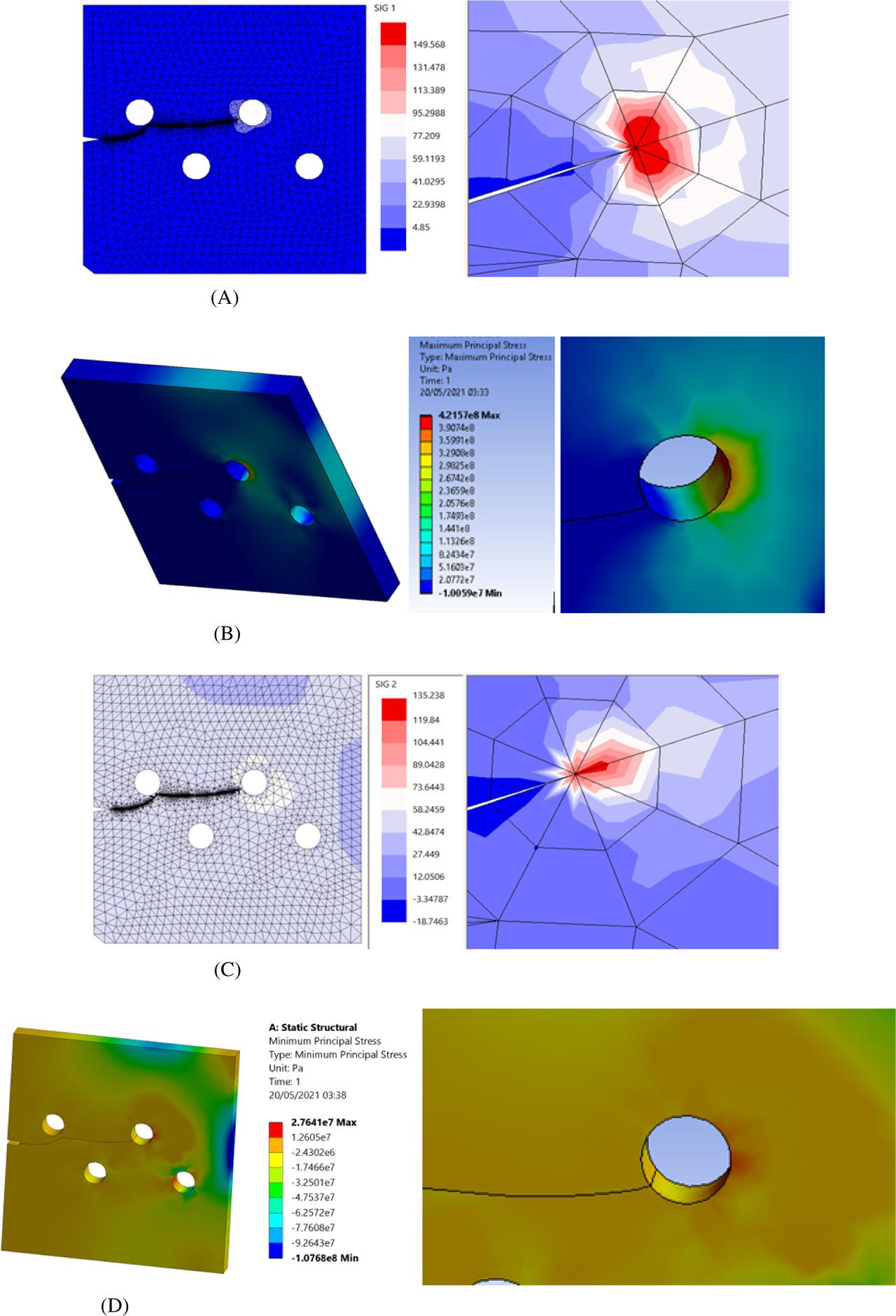Crack growth trajectory (A) maximum principal stress (developed program), (B) maximum principal stress (Ansys), (C) minimum principal stress (developed program), (D) minimum principal stress (Ansys). All units in MPa.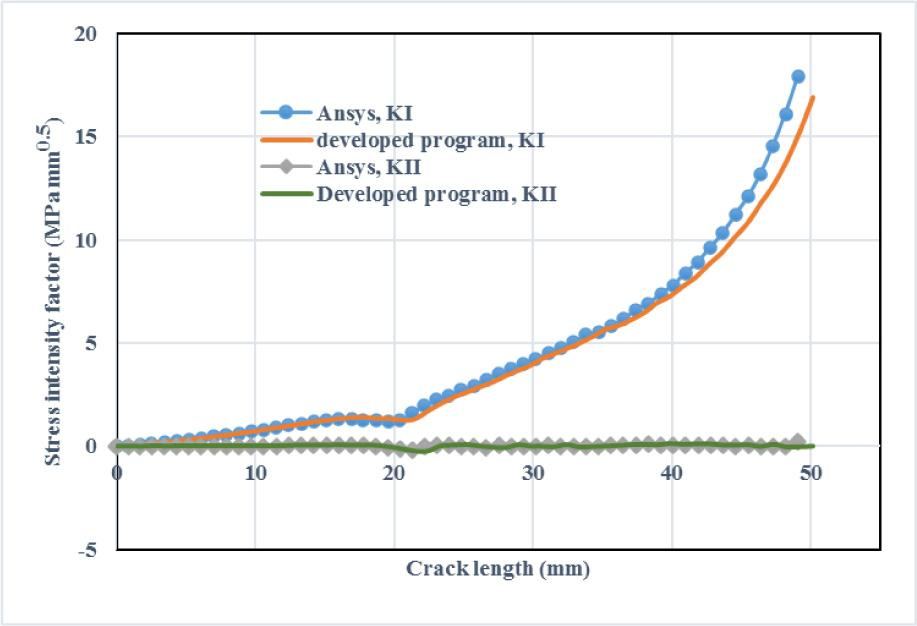KI and KII for cracked plate with four holes.

Figure 9 shows the comparison for the stress contour distribution in y direction between the developed program results, Ansys results, and the results obtained by Knowles and Sternberg  using FMM boundary element method.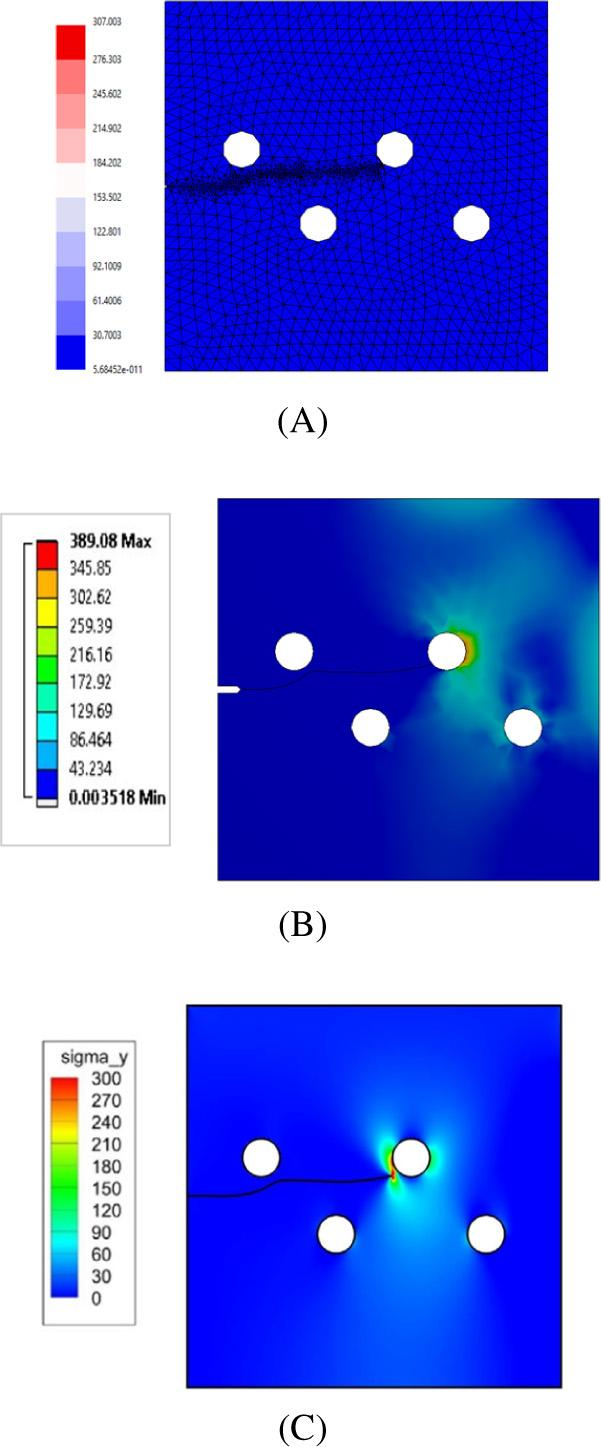Comparison for the stress contour distribution in y direction (A) developed program, (B) Ansys, and (C) FMM BEM . All units in MPa. FMM, fast multipole method.
Three holes single edge cracked plate

A more complex mixed-mode crack growth problem was investigated to illustrate the performance of the developed program to represent the propagation of cracks from beginning notches in a three holes single edge cracked plate and precisely prognosticate the values of SIFs. The geometry of three holes single edge cracked plate, and its final adaptive mesh, is presented in Figure 10. This case was investigated for the first time by Ingraffea and Grigoriu . The results revealed that small changes in the crack path's initial position significantly impacted the results. As a benchmark for numerical models, this example is commonly used to assess the system's predictive abilities. As indicated in Table 2, the geometry analyzed has 2L = 508 mm, width = 203.2 mm, and thickness = 12.7 mm, with two alternative configurations depending on the initial crack length and position. The initial meshes for this geometry, generated by the developed program and Ansys before starting the simulation, are shown in Figure 11.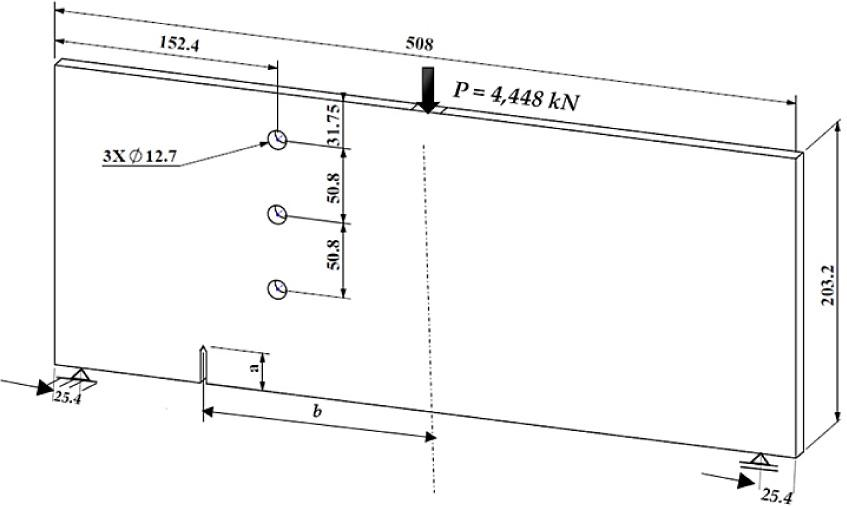Geometry and boundary condition of the three holes’ single edge crack plate.

Configurations of the three holes’ single edge cracked plate

Specimen No. Length of crack, a (mm) Crack position, b (mm)
1 25.4 152.4
2 38.1 127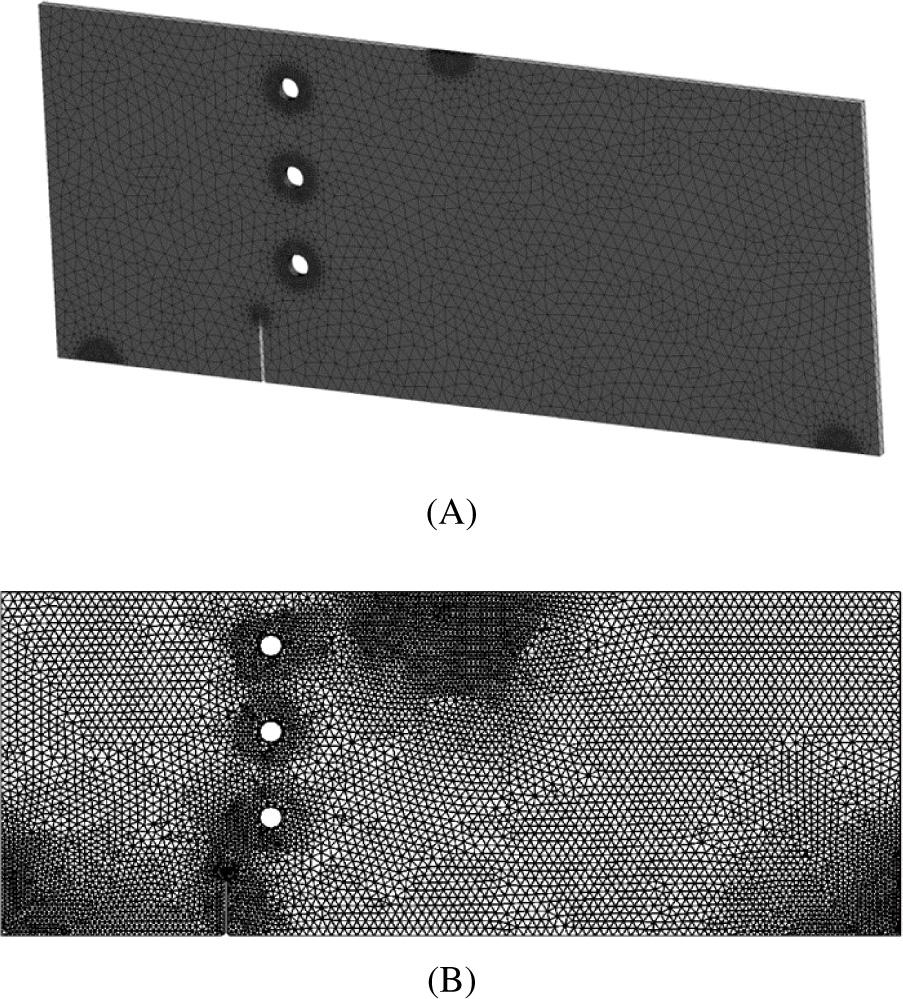Initial mesh of the three holes’ single edge cracked plate in (A) Ansys, (B) developed program.

The length of the crack in the first configurations is ao = 25.4 mm, and it is positioned at a distance of b = 152.4 mm from the mid-length of the beam. The second configuration differs from the first by having an initial crack length of ao = 38.1 mm, and it is positioned at a distance of b = 127 mm. The specimen is subjected to a cyclic point load at the upper mid-span position with a force of 4,448 kN. The material properties are shown in Table 3.

Mechanical properties of the three holes single edge cracked plate 

Properties Metric units value
Modulus of elasticity, E 205 GPa
Poisson's ratio, υ 0.3
Yield strength, σy 516 MPa
Fracture toughness, KIC 730 MPa $m$ \sqrt m
Threshold SIF, Δkth 80 MPa $mm$ \sqrt {\rm mm}
Paris’ law coefficient, C 1.2 × 10−11
Paris law exponent, m 3

Specimens are constrained to all degrees of freedom from the left pin position, similar to the experimental specimen. In contrast, the correct pin only has a first degree of freedom of translation UX. For the first configuration of specimen number one, the simulated crack propagation using the developed program and Ansys software is shown in Figure 12 with an identical crack growth trajectory. The crack growth trajectory goes between the lower and middle holes in this specimen and approaches the middle hole from the right side. It implies that the shear stress intensity factor (KII) surrounding the holes has significantly increased, forcing the crack step-sizes to be decreased.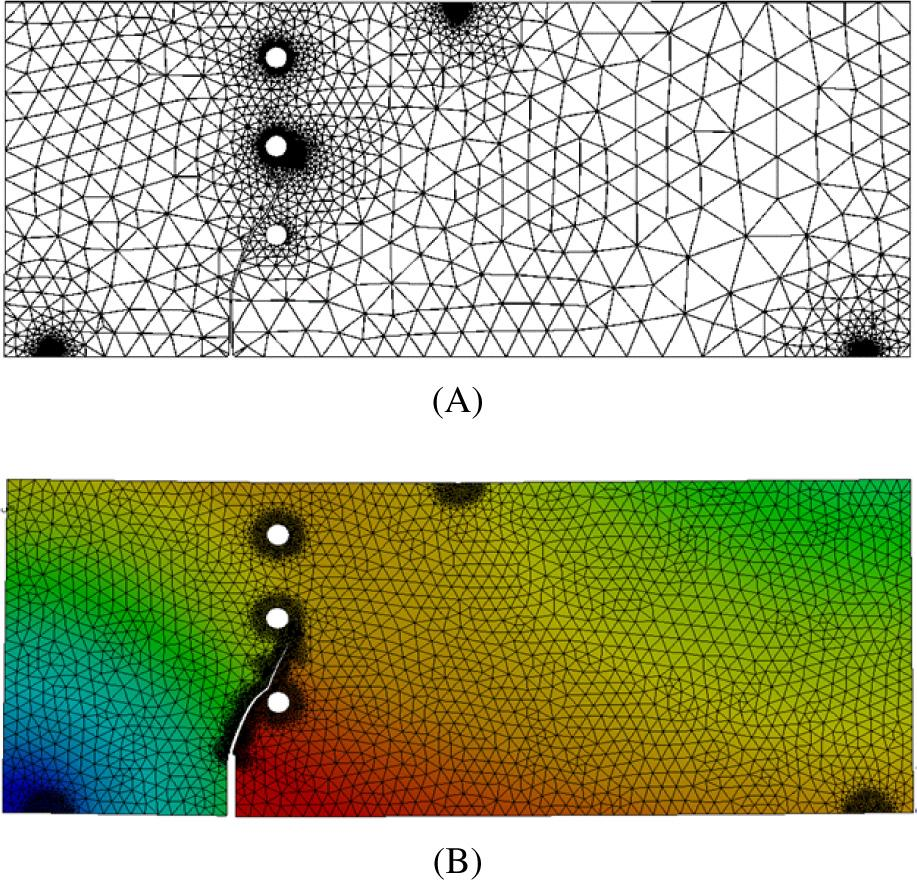Predicted crack growth path for specimen 1, (A) developed program, (B) Ansys.

The crack trajectory using the developed program and Ansys ends up in excellent agreement with experimental data presented in the literature [47, 48], numerical results using the coupled extended meshfree-smoothed meshfree method presented by Knowles and Sternberg , and XFEM results obtained by Zhang and Tabiei . Numerical results obtained by Kanth et al.  using a polygonal XFEM with numerical integration as shown in Figure 13 A, B, C, D, E, and F, respectively.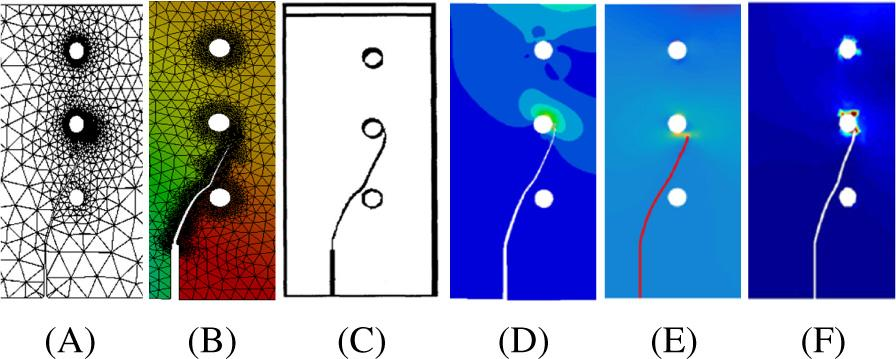Specimen 1, crack growth trajectory (A) Ansys simulation (B) developed program (C) experimental , (D) numerical results , (E) numerical results , (F) numerical results .

The results of this simulation for dimensionless stress factors by using the developed program and Ansys are shown in Figure 14, which are almost identical to each other. As can be seen in this figure, the SIFs increase in magnitude as the crack approaches the hole, demonstrating the hole's influence on the crack trajectory. For the second configuration of specimen number two, the simulated crack propagation using the developed program and Ansys software is shown in Figure 15 with an almost identical crack growth trajectory. The crack in this specimen propagates above the lower hole to the middle hole's left side.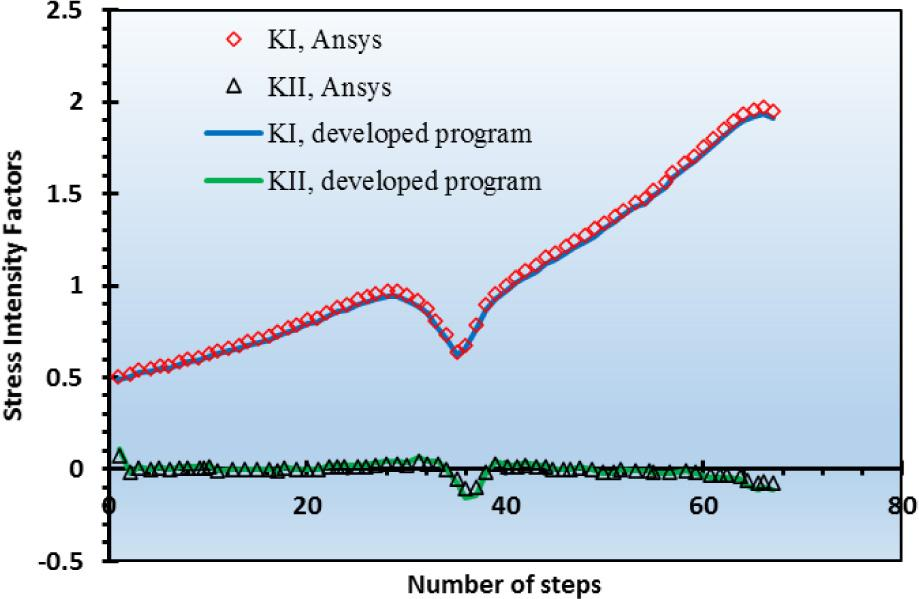Dimensionless SIFs for specimen 1. SIFs, stress intensity factors.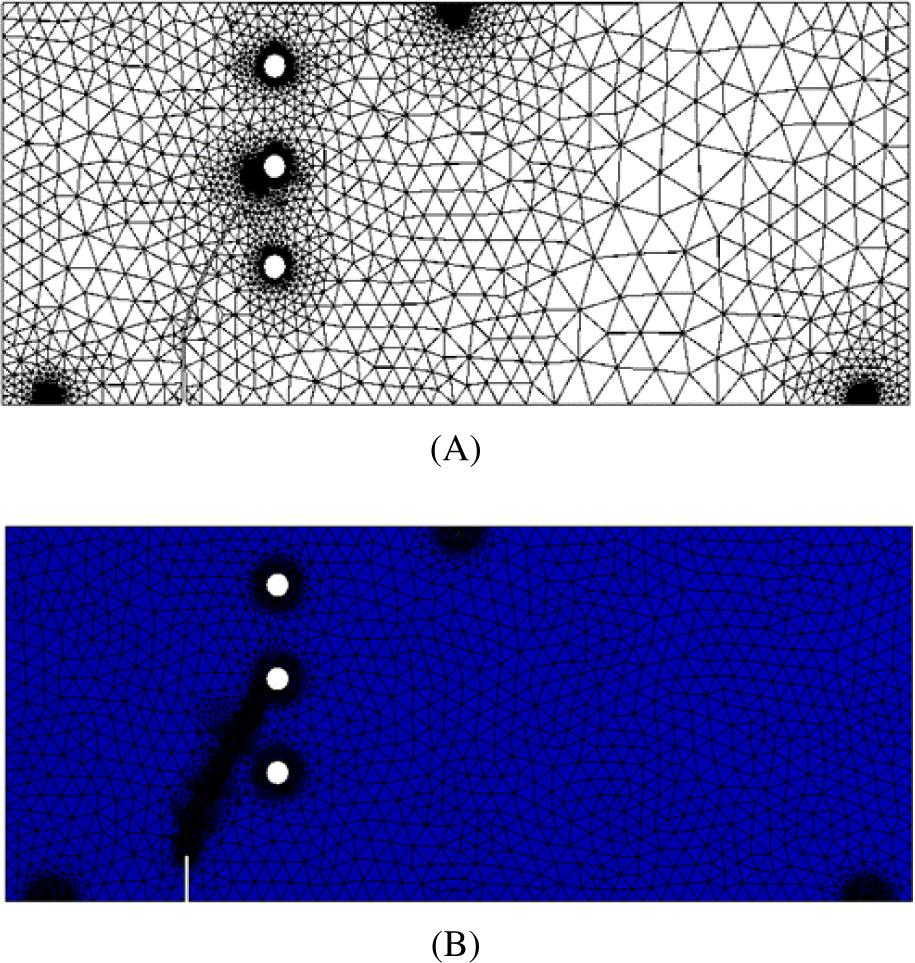Predicted crack growth path for specimen 2, (A) developed program, (B) Ansys.

Figure 16 depicts the direction of crack growth for specimen 2, which was compared to the experimental crack trajectory performed in the literature [47, 48] and the numerical findings utilizing the coupled expanded meshfree-smoothed mesh-free technique provided by Knowles and Sternberg , as illustrated in Figure 16 A, B, C, and D respectively.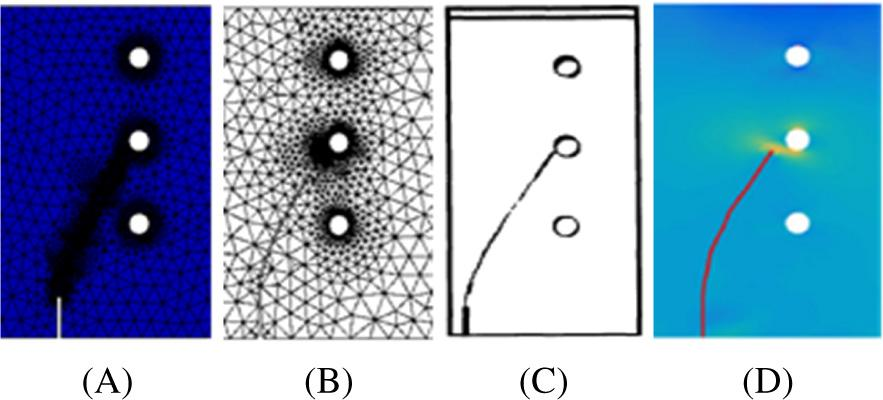Specimen 1, crack growth trajectory, (A) Ansys simulation, (B) developed program, (C) experimental [53, 55], (D) numerical results .

The predicted values of the two modes of SIFs in the developed program and Ansys are depicted in Figure 17 with excellent agreement. As observed in the figure, the lower hole has no influence on the crack growth direction, since the initial crack was far from the lower hole, while the middle hole has a stronger influence, attracting the crack to sink into the hole.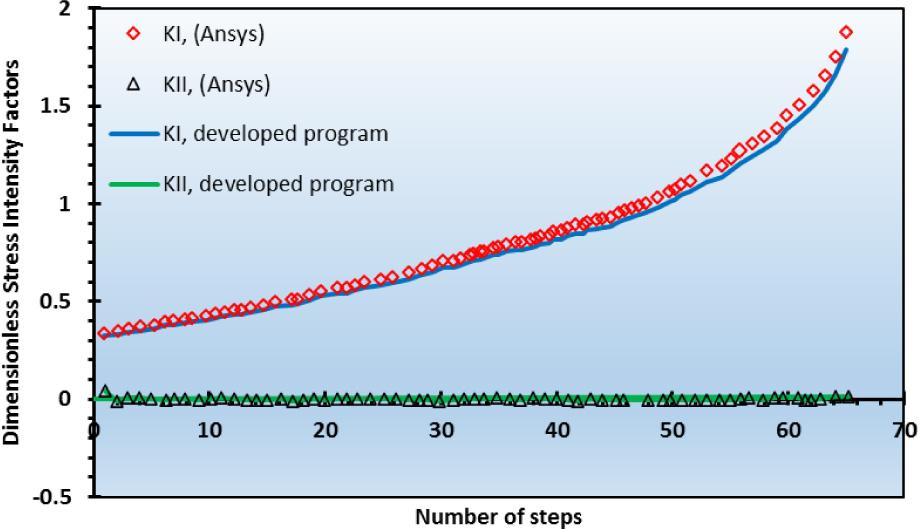Dimensionless SIFs for specimen 2. SIFs, stress intensity factors.
Conclusions

Regarding the simulation results of two- and three-dimensional analysis using the developed program and Ansys software, a satisfactory agreement was obtained between numerical and experimental crack propagation growth trajectories for the simulated specimens. However, for the first geometry there was a differential between the 2D and 3D KI values that were related to the mesh density variation. Both software's predicted fatigue life was based on Paris law, and all findings were in excellent agreement with each other. The presence of the hole in the plate influences the crack. It alters the crack trajectory to the hole. Based on the position of the hole, the crack growth trajectory will either deviate from the hole, which is known as the missed hole phenomena, or grow toward the hole and sink within, which is known as the sink in the hole phenomena. The two-dimensional analysis takes less computational time than the three-dimensional analysis and produces a fine mesh similar to the three-dimensional one due to available computational capabilities.

The three-dimensional simulation is preferable to the two-dimensional simulation to visualize better stresses, strains, deformation, and many other characteristics, despite the two-dimensional representation being sufficient.

#### Configurations of the three holes’ single edge cracked plate

Specimen No. Length of crack, a (mm) Crack position, b (mm)
1 25.4 152.4
2 38.1 127

#### Mechanical properties of aluminium 7075-T6 

Property Value in metric unit
Modulus of elasticity, E 72 GPa
Poisson's ratio, υ 0.33
Yield strength, σy 469 MPa
Ultimate strength, σu 538 MPa
Fracture toughness, KIC 3,288.76 MPa mm \sqrt {mm}

#### Mechanical properties of the three holes single edge cracked plate 

Properties Metric units value
Modulus of elasticity, E 205 GPa
Poisson's ratio, υ 0.3
Yield strength, σy 516 MPa
Fracture toughness, KIC 730 MPa m \sqrt m
Threshold SIF, Δkth 80 MPa mm \sqrt {\rm mm}
Paris’ law coefficient, C 1.2 × 10−11
Paris law exponent, m 3

Al Laham S, Branch SI. Stress intensity factor and limit load handbook. Gloucester, Volume 3. UK: British Energy Generation Limited; 1998. Al LahamS BranchSI Stress intensity factor and limit load handbook 3 Gloucester, UK British Energy Generation Limited 1998 Search in Google Scholar

Tada H, Paris PC, Irwin GR, Tada H. The stress analysis of cracks handbook, Volume 130. New York TadaH ParisPC IrwinGR TadaH The stress analysis of cracks handbook 130 New York Search in Google Scholar

Sih, G.; Liebowitz, H. Mathematical Fundamentals. In Fracture, Academic Press New York: 1968; Vol. 2, pp. 67–190. SihG. LiebowitzH Mathematical Fundamentals In Fracture Academic Press New York 1968 2 67 190 Search in Google Scholar

Hellan K. Introduction to fracture mechanics. McGraw-Hill; New York, 1985. HellanK Introduction to fracture mechanics McGraw-Hill New York 1985 Search in Google Scholar

Barsom J, Rolfe S. Fracture and fatigue in structure: Application of fracture mechanics. Philadelphia, PA: American Society for Testing and Materials; 1999. BarsomJ RolfeS Fracture and fatigue in structure: Application of fracture mechanics Philadelphia, PA American Society for Testing and Materials 1999 10.1520/MNL41-3RD-EB Search in Google Scholar

Hasan, S.; Akhtar, N. Dugdale model for three equal collinear straight cracks: An analytical approach. Theoretical and Applied Fracture Mechanics 2015, 78, 40–50. HasanS. AkhtarN Dugdale model for three equal collinear straight cracks: An analytical approach Theoretical and Applied Fracture Mechanics 2015 78 40 50 10.1016/j.tafmec.2015.04.002 Search in Google Scholar

Hasan, S.; Akhtar, N. Mathematical model for three equal collinear straight cracks: A modified Dugdale approach. Strength, Fracture and Complexity 2015, 9, 211–232. HasanS. AkhtarN. Mathematical model for three equal collinear straight cracks: A modified Dugdale approach Strength, Fracture and Complexity 2015 9 211 232 10.3233/SFC-160189 Search in Google Scholar

Kumar S, Singh I, Mishra B, Singh A. New enrichments in XFEM to model dynamic crack response of 2-D elastic solids. Int J Impact Eng. 2016;87:198–211. KumarS SinghI MishraB SinghA New enrichments in XFEM to model dynamic crack response of 2-D elastic solids Int J Impact Eng 2016 87 198 211 10.1016/j.ijimpeng.2015.03.005 Search in Google Scholar

Pandey V, Singh I, Mishra B, Ahmad S, Rao AV, Kumar V. A new framework based on continuum damage mechanics and XFEM for high cycle fatigue crack growth: ASME Press; 2000. PandeyV SinghI MishraB AhmadS RaoAV KumarV A new framework based on continuum damage mechanics and XFEM for high cycle fatigue crack growth ASME Press 2000 Search in Google Scholar

Alshoaibi AM, Fageehi YA. 2D finite element simulation of mixed mode fatigue crack propagation for CTS specimen. J Mater Res Technol. 2020;9:7850–61. AlshoaibiAM FageehiYA 2D finite element simulation of mixed mode fatigue crack propagation for CTS specimen J Mater Res Technol 2020 9 7850 61 10.1016/j.jmrt.2020.04.083 Search in Google Scholar

Li X, Li H, Liu L, Liu Y, Ju M, Zhao J. Investigating the crack initiation and propagation mechanism in brittle rocks using grain-based finite-discrete element method. Int J Rock Mech Min Sci. 2020;127:104219. LiX LiH LiuL LiuY JuM ZhaoJ Investigating the crack initiation and propagation mechanism in brittle rocks using grain-based finite-discrete element method Int J Rock Mech Min Sci 2020 127 104219 10.1016/j.ijrmms.2020.104219 Search in Google Scholar

Leclerc W, Haddad H, Guessasma M. On the suitability of a discrete element method to simulate cracks initiation and propagation in heterogeneous media. Int J Solids Struct. 2017;108:98–114. LeclercW HaddadH GuessasmaM On the suitability of a discrete element method to simulate cracks initiation and propagation in heterogeneous media Int J Solids Struct 2017 108 98 114 10.1016/j.ijsolstr.2016.11.015 Search in Google Scholar

Shao Y, Duan Q, Qiu S. Adaptive consistent element-free Galerkin method for phase-field model of brittle fracture. Comput Mech. 2019;64:741–67. ShaoY DuanQ QiuS Adaptive consistent element-free Galerkin method for phase-field model of brittle fracture Comput Mech 2019 64 741 67 10.1007/s00466-019-01679-2 Search in Google Scholar

Kanth SA, Harmain G, Jameel A. Modeling of nonlinear crack growth in steel and aluminum alloys by the element free galerkin method. Mater Today Proc. 2018;5:18805–14. KanthSA HarmainG JameelA Modeling of nonlinear crack growth in steel and aluminum alloys by the element free galerkin method Mater Today Proc 2018 5 18805 14 10.1016/j.matpr.2018.06.227 Search in Google Scholar

Huynh HD, Nguyen MN, Cusatis G, Tanaka S, Bui TQ. A polygonal XFEM with new numerical integration for linear elastic fracture mechanics. Eng Fract Mech. 2019;213:241–63. HuynhHD NguyenMN CusatisG TanakaS BuiTQ A polygonal XFEM with new numerical integration for linear elastic fracture mechanics Eng Fract Mech 2019 213 241 63 10.1016/j.engfracmech.2019.04.002 Search in Google Scholar

Surendran M, Natarajan S, Palani G, Bordas SP. Linear smoothed extended finite element method for fatigue crack growth simulations. Eng Fract Mech. 2019;206:551–64. SurendranM NatarajanS PalaniG BordasSP Linear smoothed extended finite element method for fatigue crack growth simulations Eng Fract Mech 2019 206 551 64 10.1016/j.engfracmech.2018.11.011 Search in Google Scholar

Rozumek D, Marciniak Z, Lesiuk G, Correia J. Mixed mode I/II/III fatigue crack growth in S355 steel. Procedia Struct Integr. 2017;5:896–903. RozumekD MarciniakZ LesiukG CorreiaJ Mixed mode I/II/III fatigue crack growth in S355 steel Procedia Struct Integr 2017 5 896 903 10.1016/j.prostr.2017.07.125 Search in Google Scholar

Dekker R, van der Meer F, Maljaars J, Sluys L. A cohesive XFEM model for simulating fatigue crack growth under mixed-mode loading and overloading. Int J Numer Methods Eng. 2019;118:561–77. DekkerR van der MeerF MaljaarsJ SluysL A cohesive XFEM model for simulating fatigue crack growth under mixed-mode loading and overloading Int J Numer Methods Eng 2019 118 561 77 10.1002/nme.6026 Search in Google Scholar

Rezaei S, Wulfinghoff S, Reese S. Prediction of fracture and damage in micro/nano coating systems using cohesive zone elements. Int J Solids Struct. 2017;121:62–74. RezaeiS WulfinghoffS ReeseS Prediction of fracture and damage in micro/nano coating systems using cohesive zone elements Int J Solids Struct 2017 121 62 74 10.1016/j.ijsolstr.2017.05.016 Search in Google Scholar

Xu W, Wu X. Weight functions and strip-yield model analysis for three collinear cracks. Eng Fract Mech. 2012;85: 73–87. XuW WuX Weight functions and strip-yield model analysis for three collinear cracks Eng Fract Mech 2012 85 73 87 10.1016/j.engfracmech.2012.02.009 Search in Google Scholar

Zhang W, Tabiei A. An efficient implementation of phase field method with explicit time integration. J Appl Comput Mech. 2020;6:373–82. ZhangW TabieiA An efficient implementation of phase field method with explicit time integration J Appl Comput Mech 2020 6 373 82 Search in Google Scholar

Dirik H, Yalçinkaya T. Crack path and life prediction under mixed mode cyclic variable amplitude loading through XFEM. Int J Fatigue. 2018;114:34–50. DirikH YalçinkayaT Crack path and life prediction under mixed mode cyclic variable amplitude loading through XFEM Int J Fatigue 2018 114 34 50 10.1016/j.ijfatigue.2018.04.026 Search in Google Scholar

Demir O, Ayhan AO, İriç S. A new specimen for mixed mode-I/II fracture tests: Modeling, experiments and criteria development. Eng Fract Mech. 2017;178:457–76. DemirO AyhanAO İriçS A new specimen for mixed mode-I/II fracture tests: Modeling, experiments and criteria development Eng Fract Mech 2017 178 457 76 10.1016/j.engfracmech.2017.02.019 Search in Google Scholar

Zhang R, Guo R. Determination of crack tip stress intensity factors by singular Voronoi cell finite element model. Eng Fract Mech. 2018;197:206–16. ZhangR GuoR Determination of crack tip stress intensity factors by singular Voronoi cell finite element model Eng Fract Mech 2018 197 206 16 10.1016/j.engfracmech.2018.04.042 Search in Google Scholar

Belytschko T, Black T. Elastic crack growth in finite elements with minimal remeshing. Int J Numer Methods Eng. 1999;45:601–20. BelytschkoT BlackT Elastic crack growth in finite elements with minimal remeshing Int J Numer Methods Eng 1999 45 601 20 10.1002/(SICI)1097-0207(19990620)45:5<601::AID-NME598>3.0.CO;2-S Search in Google Scholar

Bergara A, Dorado J, Martin-Meizoso A, Martínez-Esnaola J. Fatigue crack propagation in complex stress fields: Experiments and numerical simulations using the Extended Finite Element Method (XFEM). Int J Fatigue. 2017;103:112–21. BergaraA DoradoJ Martin-MeizosoA Martínez-EsnaolaJ Fatigue crack propagation in complex stress fields: Experiments and numerical simulations using the Extended Finite Element Method (XFEM) Int J Fatigue 2017 103 112 21 10.1016/j.ijfatigue.2017.05.026 Search in Google Scholar

Demir O, Ayhan AO, Sedat I, Lekesiz H. Evaluation of mixed mode-I/II criteria for fatigue crack propagation using experiments and modeling. Chinese J Aeronaut 2018;31:1525–34. DemirO AyhanAO SedatI LekesizH Evaluation of mixed mode-I/II criteria for fatigue crack propagation using experiments and modeling Chinese J Aeronaut 2018 31 1525 34 10.1016/j.cja.2018.05.009 Search in Google Scholar

Sajith S, Murthy K, Robi P. Experimental and numerical investigation of mixed mode fatigue crack growth models in aluminum 6061-T6. Int J Fatigue. 2020;130:105285. SajithS MurthyK RobiP Experimental and numerical investigation of mixed mode fatigue crack growth models in aluminum 6061-T6 Int J Fatigue 2020 130 105285 10.1016/j.ijfatigue.2019.105285 Search in Google Scholar

Alshoaibi AM. Finite element procedures for the numerical simulation of fatigue crack propagation under mixed mode loading. Struct Eng Mech. 2010;35:283–99. AlshoaibiAM Finite element procedures for the numerical simulation of fatigue crack propagation under mixed mode loading Struct Eng Mech 2010 35 283 99 10.12989/sem.2010.35.3.283 Search in Google Scholar

Alshoaibi AM. Comprehensive comparisons of two and three dimensional numerical estimation of stress intensity factors and crack propagation in linear elastic analysis. Int J Integr Eng. 2019;11:45–52. AlshoaibiAM Comprehensive comparisons of two and three dimensional numerical estimation of stress intensity factors and crack propagation in linear elastic analysis Int J Integr Eng 2019 11 45 52 10.30880/ijie.2019.11.06.006 Search in Google Scholar

Fageehi YA, Alshoaibi AM. Numerical simulation of mixed-mode fatigue crack growth for compact tension shear specimen. Adv Mater Sci Eng. 2020;1–14. https://doi.org/10.1155/2020/5426831 FageehiYA AlshoaibiAM Numerical simulation of mixed-mode fatigue crack growth for compact tension shear specimen Adv Mater Sci Eng 2020 1 14 https://doi.org/10.1155/2020/5426831 10.1155/2020/5426831 Search in Google Scholar

Chen H, Wang Q, Zeng W, Liu G, Sun J, He L, et al. Dynamic brittle crack propagation modeling using singular edge-based smoothed finite element method with local mesh rezoning. Eur J Mech A Solids 2019;76:208–23. ChenH WangQ ZengW LiuG SunJ HeL Dynamic brittle crack propagation modeling using singular edge-based smoothed finite element method with local mesh rezoning Eur J Mech A Solids 2019 76 208 23 10.1016/j.euromechsol.2019.04.010 Search in Google Scholar

Gomes G, Miranda AC. Analysis of crack growth problems using the object-oriented program bemcracker2D. Frattura ed Integrità Strutturale 2018;12:67–85. GomesG MirandaAC Analysis of crack growth problems using the object-oriented program bemcracker2D Frattura ed Integrità Strutturale 2018 12 67 85 10.3221/IGF-ESIS.45.06 Search in Google Scholar

Fageehi YA, Alshoaibi AM. Nonplanar crack growth simulation of multiple cracks using finite element method. Adv Mater Sci Eng. 2020; 1–12. FageehiYA AlshoaibiAM Nonplanar crack growth simulation of multiple cracks using finite element method Adv Mater Sci Eng 2020 1 12 10.1155/2020/8379695 Search in Google Scholar

Alshoaibi AM. Numerical modeling of crack growth under mixed-mode loading. Appl Sci. 2021;11:2975. AlshoaibiAM Numerical modeling of crack growth under mixed-mode loading Appl Sci 2021 11 2975 10.3390/app11072975 Search in Google Scholar

Paris P, Erdogan F. A critical analysis of crack propagation laws; J. Basic Eng. Dec 1963, 85(4): 528–53337. ParisP ErdoganF A critical analysis of crack propagation laws J. Basic Eng. Dec 1963 85 4 528 53337 10.1115/1.3656900 Search in Google Scholar

Coffin L. Cyclic deformation and fatigue of metals. Fatigue and Endurance of Metals [Russian translation], Moscow; 1963. 257–72. CoffinL Cyclic deformation and fatigue of metals Fatigue and Endurance of Metals [Russian translation] Moscow 1963 257 72 Search in Google Scholar

Wöhler A. Versuche zur Ermittlung der auf die Eisenbahnwagenachsen einwirkenden Kräfte und die Widerstandsfähigkeit der Wagen-Achsen. Zeitschrift für Bauwesen. 1860;10:583–614. WöhlerA Versuche zur Ermittlung der auf die Eisenbahnwagenachsen einwirkenden Kräfte und die Widerstandsfähigkeit der Wagen-Achsen Zeitschrift für Bauwesen 1860 10 583 614 Search in Google Scholar

Bjørheim F. Practical comparison of crack meshing in ANSYS mechanical APDL 19.2. Norway: University of Stavanger; 2019. BjørheimF Practical comparison of crack meshing in ANSYS mechanical APDL 19.2 Norway University of Stavanger 2019 Search in Google Scholar

Erdogan F, Sih G. On the crack extension in plates under plane loading and transverse shear. J Basic Eng. 1963;85:519–525. ErdoganF SihG On the crack extension in plates under plane loading and transverse shear J Basic Eng 1963 85 519 525 10.1115/1.3656897 Search in Google Scholar

Hussain M, Pu S, Underwood J. Strain energy release rate for a crack under combined mode I and mode II. In Proceedings of the Fracture analysis: Proceedings of the 1973 national symposium on fracture mechanics, Part II; West Conshohocken, PA, 1974. HussainM PuS UnderwoodJ Strain energy release rate for a crack under combined mode I and mode II In Proceedings of the Fracture analysis: Proceedings of the 1973 national symposium on fracture mechanics, Part II West Conshohocken, PA 1974 Search in Google Scholar

Nuismer R. An energy release rate criterion for mixed mode fracture. Int J Fract. 1975;11:245–50. NuismerR An energy release rate criterion for mixed mode fracture Int J Fract 1975 11 245 50 10.1007/BF00038891 Search in Google Scholar

Lee Y-L, Pan J, Hathaway R, Barkey M. Fatigue testing and analysis: Theory and practice, Volume 13. Burlington, Mass.: Butterworth-Heinemann, 2005. LeeY-L PanJ HathawayR BarkeyM Fatigue testing and analysis: Theory and practice 13 Burlington, Mass. Butterworth-Heinemann 2005 Search in Google Scholar

Irwin GR. Analysis of stresses and strains near the end of a crack transversing a plate. Trans ASME Ser E J Appl Mech. 1957;24:361–4. IrwinGR Analysis of stresses and strains near the end of a crack transversing a plate Trans ASME Ser E J Appl Mech 1957 24 361 4 10.1115/1.4011547 Search in Google Scholar

Bashiri AH, Alshoaibi AM. Adaptive finite element prediction of fatigue life and crack path in 2D structural components. Metals. 2020;10:1316. BashiriAH AlshoaibiAM Adaptive finite element prediction of fatigue life and crack path in 2D structural components Metals 2020 10 1316 10.3390/met10101316 Search in Google Scholar

Rice JR. A path independent integral and the approximate analysis of strain concentration by notches and cracks. J Appl Mech. 1968;35:379–86. RiceJR A path independent integral and the approximate analysis of strain concentration by notches and cracks J Appl Mech 1968 35 379 86 10.21236/AD0653716 Search in Google Scholar

Alshoaibi AM. Finite element simulation of fatigue life estimation and crack path prediction of two dimensional structures components. HKIE Trans. 2013;15:1–6. AlshoaibiAM Finite element simulation of fatigue life estimation and crack path prediction of two dimensional structures components HKIE Trans 2013 15 1 6 10.1080/1023697X.2008.10668103 Search in Google Scholar

Alshoaibi AM. An adaptive finite element framework for fatigue crack propagation under constant amplitude loading. Int J Appl Sci Eng. 2015;13:261–70. AlshoaibiAM An adaptive finite element framework for fatigue crack propagation under constant amplitude loading Int J Appl Sci Eng 2015 13 261 70 Search in Google Scholar

Alshoaibi AM. A two dimensional simulation of crack propagation using adaptive finite element analysis. J Comput Appl Mech. 2018;49:335. AlshoaibiAM A two dimensional simulation of crack propagation using adaptive finite element analysis J Comput Appl Mech 2018 49 335 Search in Google Scholar

Alshoaibi AM, Hadi M, Ariffin A. Two-dimensional numerical estimation of stress intensity factors and crack propagation in linear elastic analysis. Struct Durability Health Monit. 2007;3:15. AlshoaibiAM HadiM AriffinA Two-dimensional numerical estimation of stress intensity factors and crack propagation in linear elastic analysis Struct Durability Health Monit 2007 3 15 Search in Google Scholar

Knowles JK, Sternberg E. On a class of conservation laws in linearized and finite elastostatics. California Institute of Technology, Pasadena Division of Engineering and Applied Science; 1971. KnowlesJK SternbergE On a class of conservation laws in linearized and finite elastostatics California Institute of Technology, Pasadena Division of Engineering and Applied Science 1971 10.1007/BF00250778 Search in Google Scholar

Liu Y, Li Y, Xie WJ. Modeling of multiple crack propagation in 2-D elastic solids by the fast multipole boundary element method. Eng Fract Mech. 2017;172:1–16. LiuY LiY XieWJ Modeling of multiple crack propagation in 2-D elastic solids by the fast multipole boundary element method Eng Fract Mech 2017 172 1 16 10.1016/j.engfracmech.2017.01.010 Search in Google Scholar

Ingraffea AR, Grigoriu M. Probabilistic fracture mechanics: A validation of predictive capability. Cornell University Ithaca, NY, Department of Structural Engineering; 1990. IngraffeaAR GrigoriuM Probabilistic fracture mechanics: A validation of predictive capability Cornell University Ithaca, NY, Department of Structural Engineering 1990 Search in Google Scholar

Ma W, Liu G, Wang W. A coupled extended meshfree – Smoothed meshfree method for crack growth simulation. Theor Appl Fract Mech. 2020;107:102572. MaW LiuG WangW A coupled extended meshfree – Smoothed meshfree method for crack growth simulation Theor Appl Fract Mech 2020 107 102572 10.1016/j.tafmec.2020.102572 Search in Google Scholar

Bittencourt T, Wawrzynek P, Ingraffea A, Sousa J. Quasi-automatic simulation of crack propagation for 2D LEFM problems. Eng Fract Mech. 1996;55:321–34. BittencourtT WawrzynekP IngraffeaA SousaJ Quasi-automatic simulation of crack propagation for 2D LEFM problems Eng Fract Mech 1996 55 321 34 10.1016/0013-7944(95)00247-2 Search in Google Scholar

• #### Growth, spectral, optical, dielectric and third order nonlinear studies on 2-amino 4-methylpyridinium salicylate single crystals

Recommended articles from Trend MD